• Call Now

1800-102-2727•

# Continuous Charge Distribution

When the charge is distributed uniformly over a conductor, it is known as a continuous charge distribution. In a continuous charge distribution, the infinite number of charges are closely packed together so that there is no space left between them. As a result, the load distribution is uninterrupted and flows continuously throughout the charging system, unlike the discrete charging system.

Three types of continuous charge distribution systems occur:
1. Linear charge distribution
2. Surface charge distribution
3. Volume charge distribution

## Linear charge distribution

The charge distributed over a conductor length is known as the linear charge distribution (commonly known as the linear charge density). It is denoted by the symbol λ (pronounced as lambda). In mathematical terms, we denote linear charge distribution dq over a length dl as:
λ = dq / dl

The unit of linear charge distribution is coulomb per meter (C/m). The charge dq is present over a small length dl. If we need to find a big amount of charge over the entire length L, we need to integrate the above equation. Therefore,
dq = λ dl

Also, electric field dE is given by,
dE = k dq / r2
Putting dq from above equation, we get,
dE = k λ dl / r2

We need to integrate the whole function as: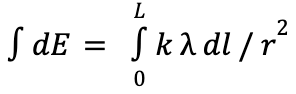to get the electric field in the linear charge distribution.
It can be simplified as: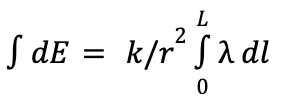## Surface charge distribution

If a uniform charge is distributed over a surface, then it is called continuous surface charge distribution. It is also known as surface charge density or charge per unit area, and is denoted by the symbol 𝜎 (pronounced sigma). Its units are coulombs per square meter (C/m2). Mathematically, surface charge density is given by:

𝜎 = dq /ds

The electric field generated by a small charge dq is denoted by dE and given as:

dE = k dq / r2

Putting the value of dq, we have,

dE = k 𝜎 ds / r2

To find the overall electric field E over the surface S produced by charge Q, we need to integrate the above equation with proper limits as: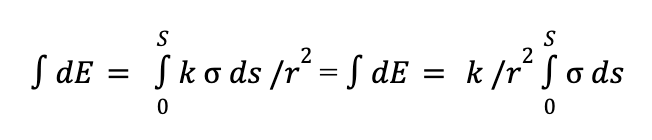## Volume charge distribution

Suppose the charge is distributed uniformly over a space or volume of any metal or even air. In that case, it is known as volume charge distribution or volume charge density or charge per unit volume. It is represented by the symbol ρ (pronounced as rho) and is denoted by coulomb per cube meter (C/m3). Mathematically, volume charge distribution is given by: ρ = dq / dv

Here, dq is a small charge present over a small volume dv. A small amount of electric field will also be generated when these charges will move from one place to another. This electric field is denoted by dE, and is given as:

dE = k dq / r2

Putting the value of dq from the above equation, we get,

dE = k ρ dv / r2

We need to integrate this equation to get electric field E over the volume V produced by charge Q as: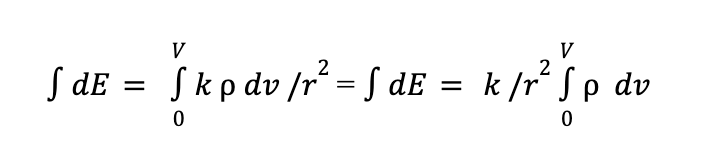## Electric field calculation

We know, different electric fields will be produced in different shapes. Whether it is a volume, a surface or even linear, different electric fields will be generated. Consider a point P outside the volume element where we need to find the magnitude of the electric field produced by charge dQ over a volume dV. Also, consider the distance between this point P and the volume element be r. Then as per Coulomb’s law, the electric field is given by: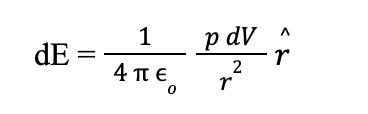Here r is the unit vector acting in the direction of the electric field produced by the charge dQ to point P. To find the entire charge over the body, we use the superposition principle. Then the electric field E is given by,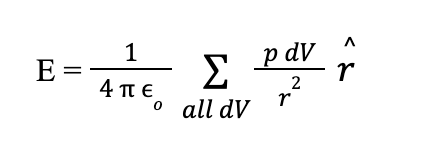## Importance of continuous charge distribution

1. For devices to get charged, appliances require continuous charge distribution so that the battery is charged uniformly.
2. For the proper functioning of home appliances, continuous charge density plays a crucial role. If the charge is non-uniform, then the appliance may not work properly.
3. However, there is no uniform charge distribution that occurs in a body. There will always be some fluctuations in the charge produced.
4. Moreover, continuous charge distribution helps store more energy by packing the charges closely to one another over a conductor.

Talk to our expert
Resend OTP Timer =
By submitting up, I agree to receive all the Whatsapp communication on my registered number and Aakash terms and conditions and privacy policy